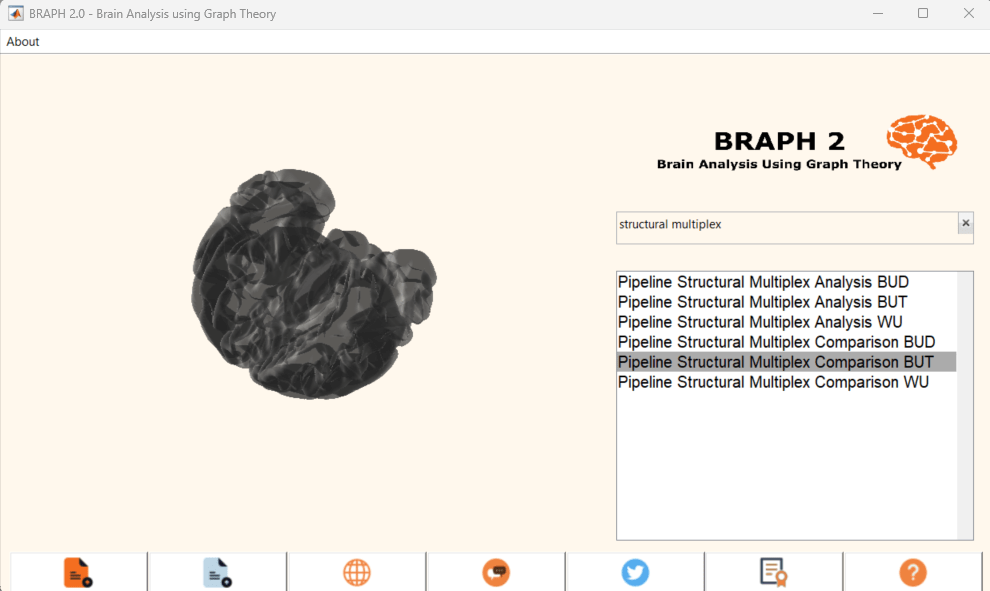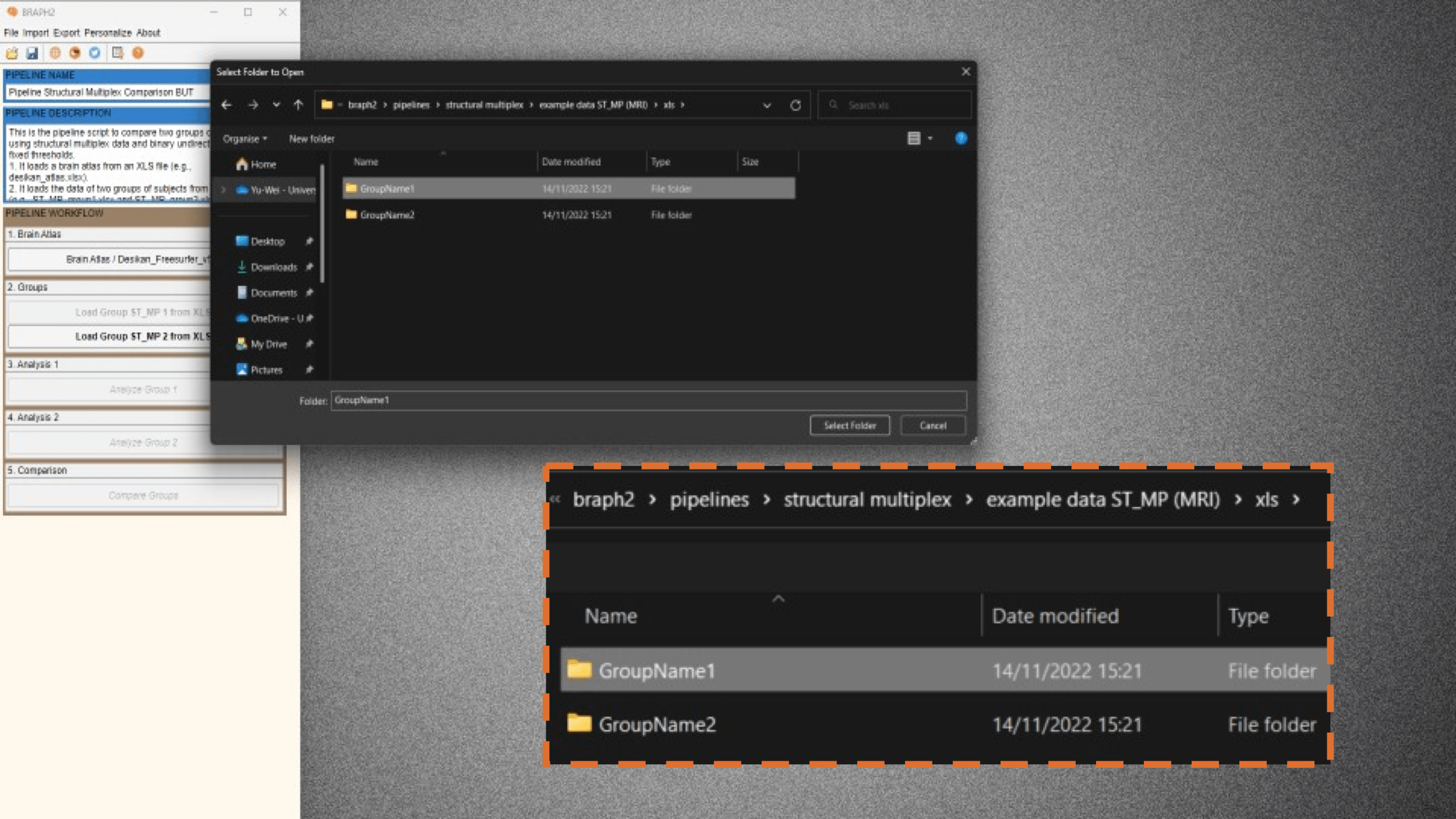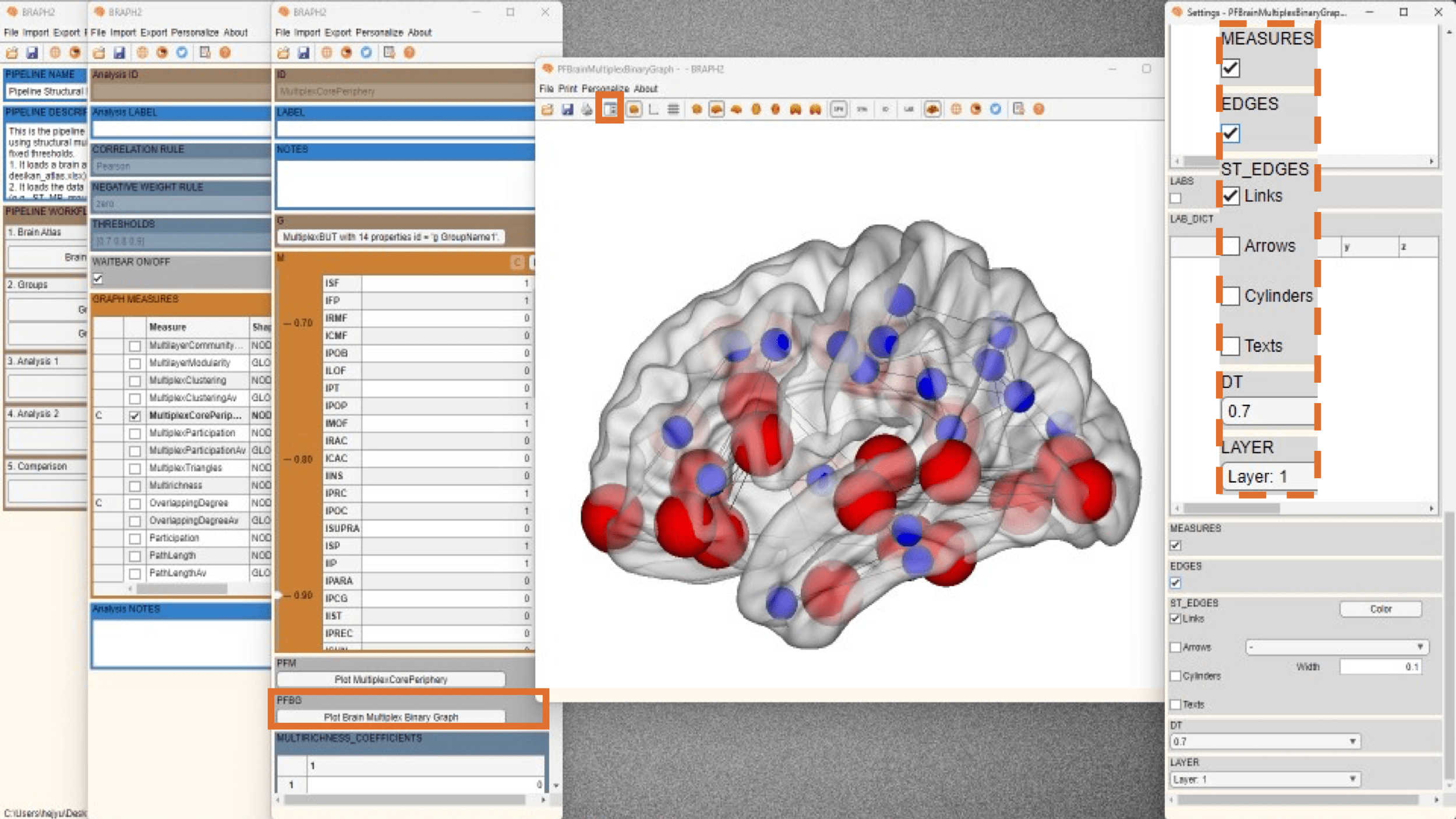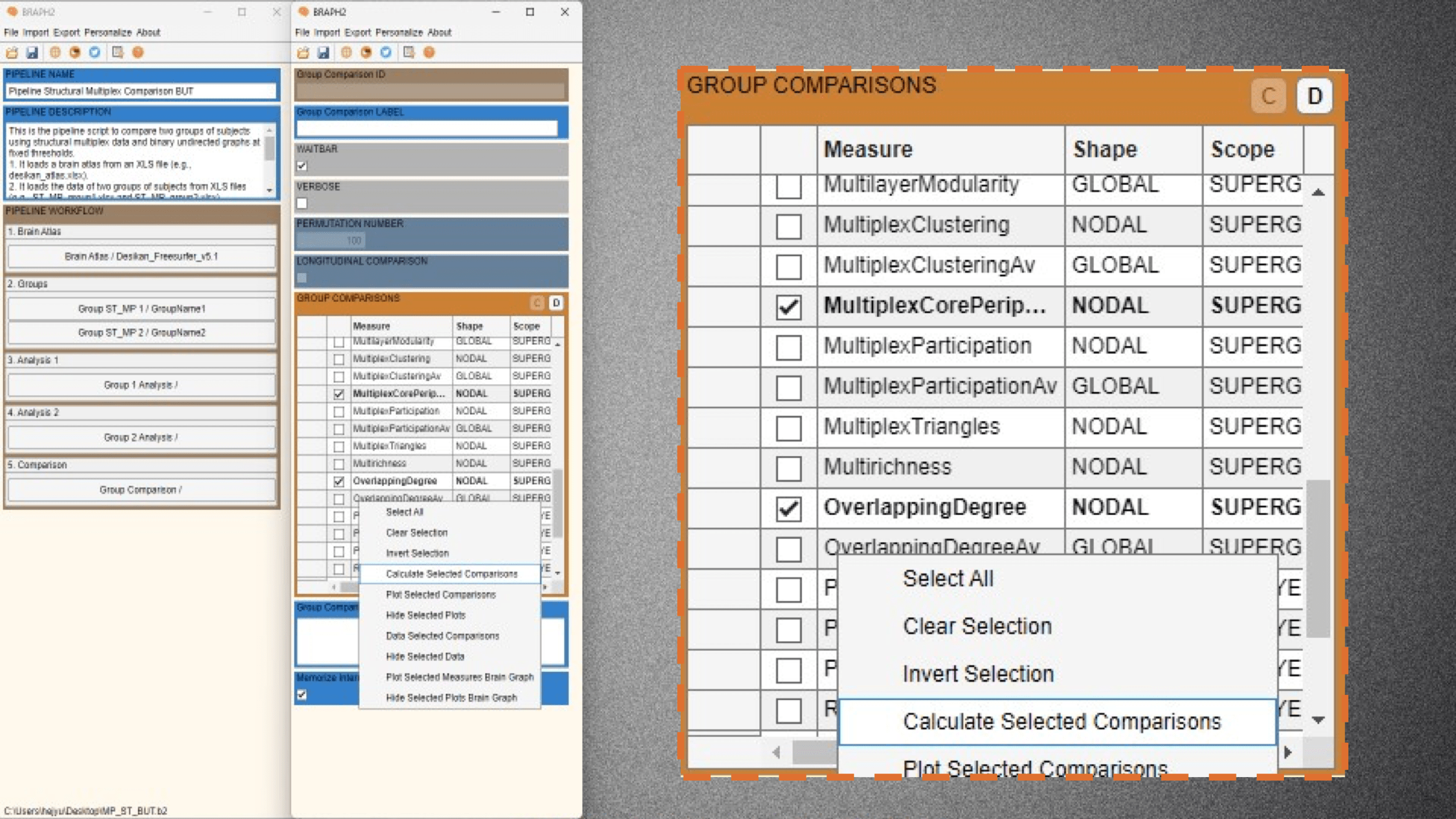# Pipeline Structural Multiplex Comparison BUT

Description:

This is the Graphical User Interface (GUI) tutorial for the pipeline that compares two groups of subjects using structural multiplex data (such as MRI and PET) and binary undirected graphs at fixed thresholds.

• Start BRAPH 2 and select the Pipeline from the main GUI

Start MATLAB, navigate to the BRAPH2 folder and run “braph2” with the following command:

>> braph2

Select the Pipeline Structural Multiplex Comparison BUT in the right menu. You can use the search field, typing structural multiplex for example (Figure 1).Figure 1. Landing GUI.The first step is to load a brain atlas from an XLS file. Press the button “Load a Brain Atlas XLS” (the only one active in the pipeline GUI, Figure 2) and then select the desikan_atlas.xlsx in the directory ./braph2/pipelines/structural multiplex/example data ST_MP (MRI) (Figure 2). Finally, to visualize the atlas press “Plot the brain atlas” and play with the surface settings if desired.

To load the data for the two groups you would like to compare press “Load Group ST_MP 1 from XLS” and select the file for subjects of Group1 which is in the directory ./braph2/pipelines/structural multiplex/example data ST (MRI)/xls/GroupName1. After, load the data for the group 2 which can be found in the directory ./braph2/pipelines/structural multiplex/example data ST (MRI)/xls/GroupName2 (Figure 3).Figure 3. From the pipeline GUI, first load data for group 1 (GroupName1) and then for group 2 (GroupName2).

• Performing the structural multiplex analysis of group 1

Analysis of the first group using structural multiplex analyses (ST) based on binary undirected graphs at fixed thresholds (BUT).

First press “Analyze Group 1” to open the interface for the analysis, where you can specify the parameters for constructing the graph from the structural data (type of correlation and what to do with the negative weights). For the case of structural data, it is possible to choose partial correlation rules with controlling for the covariates (age and sex).  Here, we set the thresholds to [0.7 0.8 0.9]. Then under GRAPHS MEASURES section, by pressing “C” and right clicking on the left of a measure to deploy the menu seen on Figure 4, select “Plot Graph” and you can check the graphs at group level. We will see an adjacency graph on the right, which is the graph from the first layer (Figure 4). If we go to settings and then to section “ST_ADJACENCY”, we can decide whether to plot the weighted or binary Graph. In this case, the adjacency matrix is already a binary graph at fixed thresholds. Moreover, if we change to layer 2 then we will see the adjacency matrix from the second layer (Figure 5).Figure 4. Plot graph. By default, we see the adjacency matrix at the first layer.Figure 5. Plot graph. By selecting layer 2, we see the adjacency matrix at the second layer.

Now it is the moment to calculate some connectivity measures for group 1. Select one measure, for example, Overlapping Degree (nodal superglobal) and Multiplex Core-Periphery (nodal superglobal) and calculate them by right clicking on the left-side of the measures list and pressing “Calculate Selected Measures” (Figure 6).Figure 6. Calculation of Overlapping Degree and Multiplex Core Periphery.

When the measure is calculated, a C appears on its left. Now we just keep the selected Multiplex Core-Periphery measure and right click on the left-side of the measures list and press “Data Selected Measures” to be able to check the results of the measure for group 1. From there, we press “Plot Brain Multiplex Graph” to visualize the results of Multiplex Core-Periphery for group 1 on a brain surface (Figure 7).

A new figure with the brain surface will open with default settings for brain region visualization. If we go to settings and scroll down in the Settings panel, we will find Measures and EDGES. By checking the boxes, the connection links will appear, and the size of all spheres will be adapted in proportion to the measure’s value, where blue colors represent peripherical nodes, and red core nodes (Figure 7).Figure 7. Selecting the check box at Measures and Edges.

Remember, in case we want to calculate a measure with different rules or parameters, this is done by going first to “Data Selected Measuresbefore calculating the measure. For more details, check the section Measures’ calculation with different parameters in the tutorial from Module 1.

• Performing the structural multiplex analysis of group 2

Analysis of the second group using the same parameters selected for the first group (structural multiplex analyses (ST) based on binarized undirected graphs at fixed thresholds (BUT)).

Follow the previous steps in the analysis of group 1 and explore some other measures for group 2.

• Comparing the two groups

To proceed with the group comparison press “Compare Groups” and the interface to select the comparison parameters will open. After setting the desired permutation number (recommended 1000 – 10000 permutations, although in this tutorial we use 100 for time constraints), press “C” under the ENSEMBLE COMPARISONS section to open the list of measures to use to compare the groups.

We select the Multiplex Core-Periphery and Overlapping Degree measures to be compared, and then right click on the left of the measure table and select “Calculate Selected Comparisons” (Figure 8). Depending on how many permutations you use it will take less or more time.Figure 8. Calculation of Group Comparison.

Once the calculations are done, you should see a C on the left of the measure to be compared. Then press “Data Selected Comparison” to show the difference values between groups and the associated p-values resulting from the permutation testing (Figure 9). Significant differences after FDR correction at the value of q = 0.05 (q level can be changed in the QVALUE) are highlighted in the table by green. We set a q value of 0.05 by default and there are many regions with significant difference in the multiplex core periphery measure (Figure 9).

We can then plot the results of this nodal measure from the comparison by pressing “Plot Brain Multiplex Graph Comparison” in the measure’s table GUI. A new figure with the brain surface will open with default settings for brain region visualization. If we scroll down in the Settings panel, we will find MEASURES, FDR SHOW, and QVAL. By checking the boxes, just the regions that are significantly different between groups after applying FDR correction at the desired level will be shown, and the rest will be hidden (Figure 9). In this case, all differences are in the same direction, multiplex core periphery is larger in group 1 than in group 2 (blue color indicates group 2 < group 1) at the threshold of 0.7.Figure 9. Regions that are significant after applying FDR at a q_value = 0.05.